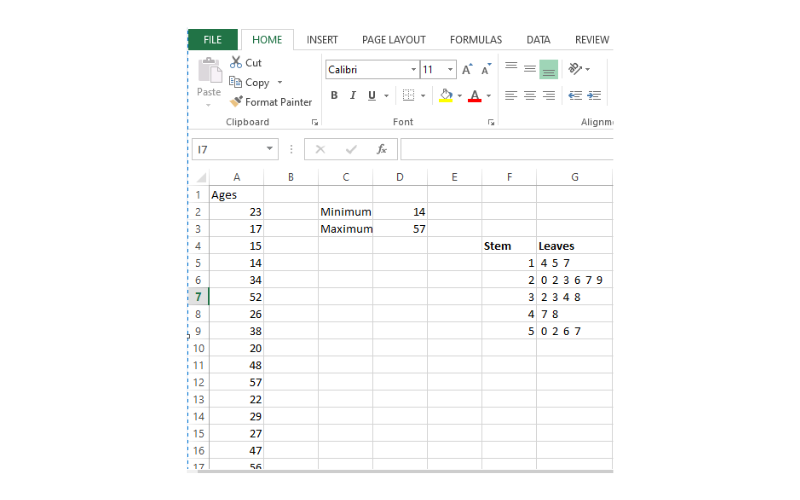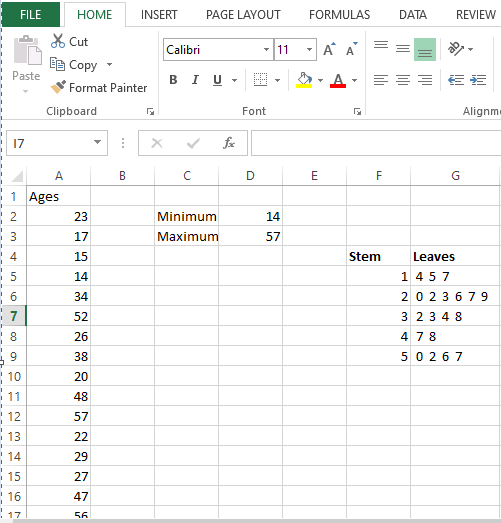# How to Create a Stem and Leaf Plot in Excel## What is a Stem and Leaf Plot?

A Stem and Leaf Plot can easily be described as a table or chart that visualizes the distribution of numbers within a specified range.

These numbers may or may not be sorted in an ascending order or descending order.

It depends on the preference of the individual plotting the stem and leaf plot.

The numbers are usually broken into smaller pieces per row, for example, it can be broken into 10s or 100s.

The individual character in a stem and leaf plot is described as follows:

• Stems: This represents the first digit or digits of the data points
• Count: This represents the number of data points with that first digit
• Leaf: The leaf represents the number farthest to the right. It is usually numbered 0-9 depending on the range of the dataset you are working with.

Stem and Leaf plots are very useful because they allow us to see the distributions across different categories of data without the use of complex mathematical computations.

A stem and leaf plot displays a series of scores in a simple and comprehensible way.

The stem usually represents the first digit of a data point, while the leaf represents the last digit.

For example, if a stem digit is eight and the leaf digit is four, the data point is 84.

## Types of data that are used with stem and leaf plots

Stem and leaf plots work best with quantitative data, especially smaller sets of data.

It is best used when you have a data set not greater than 150 data points. It works best for smaller sets of data because the plot displays the exact value of every data point within the dataset.

For example, if you are involved in a research study where you gathered the age details of about 140 participants, you may want to consider using a stem and leaf plot to chart for that data.

The following are examples of other types of data sets where a stem and leaf plot may be applied.

• Test scores for High school students of a class.
• The average weight of newborn babies.
• Temperature chart of a city over some time.
• Ages of an older group of women in a sports team.

## Creating a Stem and Leaf Plot

The objective of this tutorial is to create a layout that looks like this:To create a layout that looks like the above, the steps listed below should be followed.

To create a stem and leaf plot, you must enter the data you wish to work with.

This data may be a precise number (e.g., 34) or a distribution range (e.g., 10 – 19) such as age group, test scores, etc.

In this tutorial, we will be using the set of data below: The table below shows the Ages of 20 participants from a research study.

 Ages 23 17 15 14 34 52 26 38 20 48 57 22 29 27 47 56 50 33 32

Step Two: Determine the minimum and maximum value of your dataset.

The minimum and maximum values allow you to determine the range of your stem

1. Type Minimum and Maximum in separate Cells (In our example this is Cell C2 and C3 respectively)
2. In the cell adjoining the Minimum, enter the Minimum function by typing “=MIN” and opening parenthesis. Select the range of values you want to determine minimum value from and close parenthesis then click on the Enter key on your keyboard. The minimum value will appear
3. In the cell adjoining the Maximum, enter the Maximum function by typing “=MAX” and then open parenthesis and select the range of values you want to determine maximum value from and then close parenthesis and hit on the enter key in your keyboard. The maximum value will appearStep Three: Create the Stem and Leaf plot.

Now that we have gotten the minimum and the maximum values, we can proceed to create our stem and leaf plot as follows:

1. Navigate to where you wish to display your values and type in the heading ‘Stem’ in one cell and ‘Leaf’ in the adjacent cell.
2. Manually enter the stems based on the minimum and maximum values. For example, the minimum and maximum values for this tutorial are 14 and 57. So, we will be needing a 1, 2, 3, 4, and 5 since the maximum value starts with a 5. You can automatically fill the numbers 1-5 by highlighting the first and second and dragging down (a plus sign shows at the edge of the last highlighted column) to the last number you want.
3. Enter the formula on your leaf column as follows:

`=REPT(" 0 ",COUNTIF(`range,ref stem cell`*10+0))&REPT(" 1 ",COUNTIF(`range,ref stem cell` *10+1))&REPT(" 2 ",COUNTIF((`range,ref stem cell` *10+2))&REPT(" 3 ",COUNTIF(`(range,ref stem cell`*10+3))&REPT(" 4 ",COUNTIF(`(range,ref stem cell`*10+4))&REPT(" 5 ",COUNTIF(`(range,ref stem cell`*10+5))&REPT(" 6 ",COUNTIF(`(range,ref stem cell`*10+6))&REPT(" 7 ",COUNTIF(`(range,ref stem cell`*10+7))&REPT(" 8 ",COUNTIF(`(range,ref stem cell`*10+8))&REPT(" 9 ",COUNTIF(`(range,ref stem cell`*10+9))`

I know this formula looks long and complicated, but trust me when I say it is not. It is just repetitive

In place of range, input your dataset range and for ref stem cell, input the corresponding Stem cell you are referencing.

For our example, our leaf formula will be:

`=REPT(" 0 ",COUNTIF(\$A\$2:\$A\$20,F5*10+0))&REPT(" 1 ",COUNTIF(\$A\$2:\$A\$20,F5*10+1))&REPT(" 2 ",COUNTIF(\$A\$2:\$A\$20,F5*10+2))&REPT(" 3 ",COUNTIF(\$A\$2:\$A\$20,F5*10+3))&REPT(" 4 ",COUNTIF(\$A\$2:\$A\$20,F5*10+4))&REPT(" 5 ",COUNTIF(\$A\$2:\$A\$20,F5*10+5))&REPT(" 6 ",COUNTIF(\$A\$2:\$A\$20,F5*10+6))&REPT(" 7 ",COUNTIF(\$A\$2:\$A\$20,F5*10+7))&REPT(" 8 ",COUNTIF(\$A\$2:\$A\$20,F5*10+8))&REPT(" 9 ",COUNTIF(\$A\$2:\$A\$20,F5*10+9))`

Once you are done entering the formula in the first row of the leaf column, hit enter. Your result is as follows:Step Four: Duplicate the calculation for each row of the leaf.

To duplicate this calculation for each row, simply click on cell G5, hover over the bottom right-hand corner of the cell until a tiny plus sign appears, then double-click and drag down to G9.

This will copy the formula to the rest of the rows in the Stem-and-Leaf plot:Note that the choice of a column is entirely dependent on you.

We can verify that our results are correct by the following steps:

• Make sure the number of individual leaves matches the number of observations. In our example, we have 19 total “leaves” which matches the 19 total number of age distribution in our original dataset.
• We can also verify if our result is correct by verifying the minimum number. If your first leaf matches with minimum value in your dataset, then your result is correct. In this example, we see that the first leaf is 4 and is paired with a stem of 1 which matches the minimum number of 14 in our dataset.
• We can also verify if our result is correct by Verifying the maximum number. If the last leaf matches the maximum value in your dataset, then the result of our stem and leaf plot will be said to be correct. In this example, we see that the last leaf is 7 and when paired with a stem of 5 it matches the maximum number of 57 in our dataset.

## Why use Stem and Leaf Plot

• Just like a histogram that is turned 180 degrees on its side, a stem and leaf plot can help show the distribution of your data (as illustrated in the sections above)
• A stem and leaf plot allows us to quickly scan and easily analyze data such as calculating the mode or median of a dataset.
• It can also help us to easily scan for outliers that may be present in a wide range of a dataset in cases where numbers are sitting far beyond the typical distribution.

## Tips for using a stem and leaf plot

To use a stem and a leaf plot, it is important to take into consideration the following.

• Keeping the data sets small: When you want to use a stem and leaf plot, it is advisable to choose a small dataset. However, if you are to create your dataset, it is also best to keep your data set small. In case you have a larger data set, you should consider using a different type of chart available in Excel such as a Pie chart, Line chart, Bar of a pie, chart, etc.
• Keeping the data organized: The stem and leaf plot is easy to read when the data is organized. When each aspect of the data is organized, it makes data visualization easier and interpretation of important charts is simplified. Although ordering both the stems and leaves in order from low to high may have a positive impact on data overview, it is not always necessary to sort your data to perform a stem and leaf plot on your data.
• Finding the mean and median: As much as stem and leaf plot helps in the visualization of your dataset, considering the implementation of other statistical methods to better understand your data is not out of place. Finding the mean, or average as well as median numbers in your dataset can help you when you want to locate the number in your stem and leaf plot.

## Conclusion

In this tutorial, we have been able to establish that the stem and leaf plot is an important tool in visualizing a set of data.

We have also simplified how excel can be used to create a stem and leaf plots.

Ovo is a highly specialized Computer and Networking Expert with experience in Windows, Cisco, Microsoft Office (Excel, Word, etc) and Networking engineering. Ovo is a creative, team player that loves sharing his experience with technology with readers to follow along. He has great attention to detail when discussing various technologies, tutorials and guides.